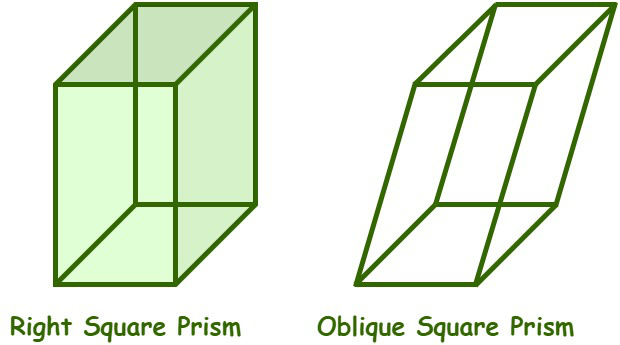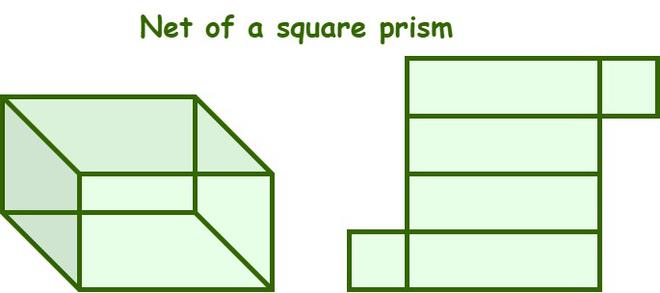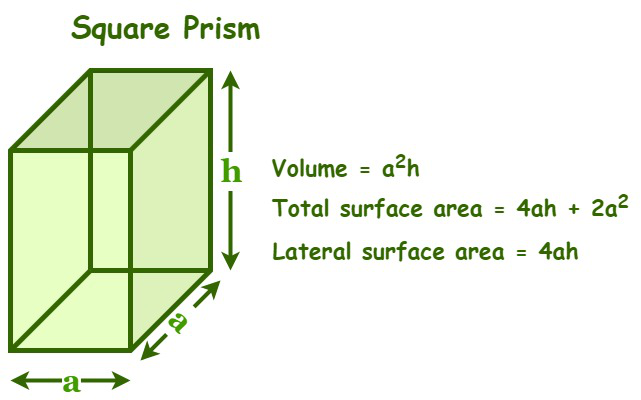Open in App
Not now

# What is a Square Prism?

• Last Updated : 18 Aug, 2022

In mathematics, a prism is a significant member of the polyhedron family. It is defined as a three-dimensional-shaped geometric structure with two identical polygons facing each other, laterally connected by rectangular or parallelogram faces. These identical polygons are the bases of a prism and can be of any shape, such as triangular, rectangular, square, pentagonal, etc. The other faces, which are called side faces or lateral faces, are either parallelograms or rectangles. We have different types of prisms based on the shape of the polygonal base, namely, triangular prisms, square prisms, rectangle prisms, Pentagonal prisms, hexagonal prisms, octagonal prisms, etc.

## Square Prism

A square prism is a prism that has a square base. It is a special case of a cuboid having square bases. It has a total of six faces, which include four rectangular faces and two parallel square bases, twelve edges, and eight vertices. The opposite faces of a square prism are parallel and congruent to each other. The rectangular faces of a square prism are called the lateral faces. There are two types of prisms based on the alignment of the square bases, i.e., a right square prism and an oblique square prism. A right square prism is a square prism whose lateral faces are perpendicular to its square bases, whereas an oblique square prism is a square prism whose lateral faces are not perpendicular to the square bases.## Square Prism Formulae

The formulas of a square prism include its formulae to determine its volume and surface areas.

## Surface area of a Square Prism

Surface area is the total area occupied by all the three-dimensional surfaces of a three-dimensional shape or object. To determine a prism’s surface area, we must calculate the areas of each of its faces, then add the resulting areas. A square prism has two types of surface areas: a lateral surface area and a total surface area.Lateral Surface Area of a square prism: The lateral surface area of a square prism is the area occupied by its lateral faces or side faces. The formula for calculating the lateral surface area of a square prism is given,

Lateral surface area of a prism = 4ah square units

Where,

“a” is the side of the square base and

“h” is the height of the square prism.

Total surface area of a square prism: The total surface area of a square prism is defined as the total area occupied by all its surfaces (four lateral faces and two square bases). The formula for calculating the total surface area of a square prism is given as,

Total surface area of the square prism = (4ah + 2a2) square units

Where’

“a” is the side of the square base, and

“h” is the height of a square prism.## Volume of a Square Prism

The volume of a square prism is defined as the amount of space enclosed by its two congruent square bases and four rectangular faces. It is measured in terms of cubic units and is equal to the product of its height and the base area.

Volume = Area of base × Height of the prism

As the bases are squares, the base area = (edge)2 = a2

By substituting the values in the above formula, we get

Volume of a square prism = a2 × h cubic units

Where,

“a” is the side of the square base and,

“h” is the height of the square prism.

## Solved Examples based on Square Prism formula

Example 1: Find the volume of a square prism whose side is 8 cm and whose height is 13 cm.

Solution:

Given data,

The length of the side of a square prism (a) = 8 cm

The height of a square prism (h) = 13 cm

We know that,

The Volume of a square prism = a2h cubic units

= (8)2 × 13

= 64 × 13

= 832 cu. cm

Thus, the volume of the given prism is 832 cu. cm.

Example 2: Calculate the surface area of a square prism whose side is 10 units and whose height is 15 units.

Solution:

Given data,

The length of the side of a square prism (a) = 10 units

The height of a square prism (h) = 15 units

We know that,

From the square prism formula, we have

Surface area of a square prism = 2a2 + 4ah square units

= 2(10)2 + (4 × 10 × 15)

= 2(100) + 600

= 200 + 600 = 800 square units

Thus, the surface area of the given prism is 800 square units.

Example 3: Find the volume of a container whose shape is a square prism with a side of 20 inches and a height of 25 inches.

Solution:

Given data,

The length of the side of a square prism (a) = 20 inches

The height of a square prism (h) = 25 inches

We know that,

The Volume of a square prism = a2h cubic units

= (20)2 × 22

= 400 × 25

= 1000 cu. in

Thus, the volume of the given prism is 1000 cu. in.

Example 4: Calculate the lateral surface area of a square prism whose side is 12 cm and whose height is 16 cm.

Solution:

Given data,

The length of the side of a square prism (a) = 12 cm

The height of a square prism (h) = 16 cm

From the square prism formula, we have

The lateral surface area of a square prism = 4ah square units

= 4 × 12 × 16 = 768 sq. cm

Thus, the lateral surface area of the given prism is 768 sq. cm.

Example 5: Determine the height of a square prism if its volume is 891 cubic units and its side length is 9 units.

Solution:

Given data,

The length of the side of a square prism (a) = 9 units

Volume (V) = 891 cubic units

Height (h) =?

From the square prism formula, we have

The Volume of a square prism = a2h cubic units

⇒ (9)2 × h = 891

⇒ 81h = 891

⇒ h = 891/81 = 11 units

Hence, the height of a square prism is 11 units.

Example 6: Determine the side length of a square prism if its lateral surface area is 660 sq. cm and its height is 15 cm.

Solution:

Given data,

The height of a square prism (h) = 15 cm

The lateral surface area = 660 sq. cm

The length of the side of a square prism (a) =?

From the square prism formula, we have

The lateral surface area of a square prism = 4ah square units

⇒ 4 × a × 15 = 660

⇒ 60a = 660

⇒ a = 660/60 = 11 cm.

Hence, the side length of the given prism is 660 sq. cm.

## FAQs based on Square Prism

Question 1: What is a square prism?

A square prism is a prism that has its base-faces in the shape of square and the other four faces are usually in the shape of rectangular. It a three-dimensional shape which closely resembles cuboidal shape.

Question 2: What are the formulas for Square prism?

Square prism has below mentioned two main formulas.

Surface area of a square prism = 2a2 + 4ah

Volume of a square prism = a2h

where,

a side-length and

h is the height of prism.

Question 3: Are all the cubes can be considered a square prism?

Yes, all the cubes are actually square prisms, but opposite is not true i.e. not all the square prisms are cubes.

Question 4: How many faces do a square prism has?

A square prism has 6 faces in total. It is similar to cube which also has six faces.

Question 5: What are the types of the square prism?